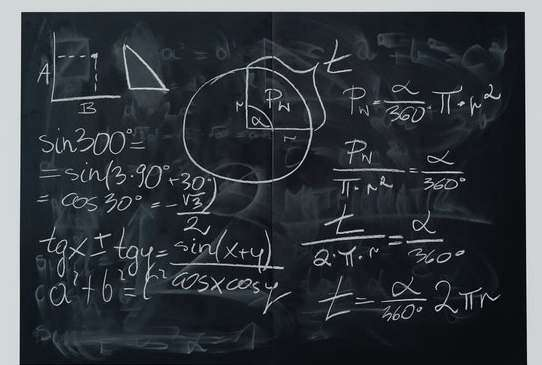# Matrix Form to System of Equations Calculator

Instructions: Use this online calculator to get a system of linear equations from its matrix representation, showing all the steps. First, click on one of the buttons below to specify the dimension of the matrix representation, then you need to specify $$A$$ and $$b$$.

For each of the matrix and vector, click on the first cell and type the value, and move around the matrix by pressing "TAB" or by clicking on the corresponding cells, to define ALL the matrix values.

$$A$$ = \begin{bmatrix} & \\ & \end{bmatrix}

$$b$$ = \begin{bmatrix} \\ \end{bmatrix}

Often times you will have a system in matrix form, with $$Ax = b$$ and you will want to actually express the matrix form into the regular linear equation form, just to see the equations in a more clear way.

If you are provided with matrix form, perhaps you will like to solve the system using Cramer's Rule, or you maybe want to solve it by using the inverse method.

### Why would you pass from matrix form to system of equation forms

The two forms are completely interchangeable, but perhaps the system of equation form allows you for a more clear interpretation of the situation you are facing, particularly in cases where the setting of the linear equation is tied to real variables.### How to convert a matrix form into system of equations form

Simple. You need to take a look at the matrix $$A$$, row by row. Each row of $$A$$ corresponds to an equation. Now, each column of those rows is associated to a certain variable.

What happens when a coefficient is zero? In that case, the associated variable does not appear in the equation.